# lawcos .pdf

### File information

Original filename: lawcos.pdf
Title: 000.1 - Law of Cosines and Sines
Author: James Bonnar

This PDF 1.4 document has been generated by LaTeX with hyperref package / pdfTeX-1.40.3, and has been sent on pdf-archive.com on 28/07/2011 at 13:37, from IP address 98.144.x.x. The current document download page has been viewed 1347 times.
File size: 96 KB (5 pages).
Privacy: public file

lawcos.pdf (PDF, 96 KB)

### Document preview

000.1 - The Law of Cosines and Sines
c
2010
Treasure Trove of Mathematics

The law of cosines and the law of sines are two trigonometric equations
commonly applied to find lengths and angles in a general triangle.1

1

The Law of Cosines

Suppose we have a triangle labeled by the vertex points A, B, and C, sides
a, b and c, and angles α, β, and γ, as shown in Figure 1. In what follows,
we consider all lengths and angles to be positive quantities.
The law of cosines is used to solve a triangle for
• a third side, c, of the triangle, if two sides, a and b, and the angle γ
between them is known:
c=

q

a2 + b2 − 2ab cos γ

• the angles α, β and γ if the three sides, a, b and c are known:
b2 + c2 − a2
2bc
a2 + c2 − b2
β = arccos
2ac
a2 + b2 − c2
γ = arccos
2ab

α = arccos

• the third side a of a triangle if one knows two sides, say, b and c, and
an angle opposite one of them, say, γ:
a = b cos γ ±
1

q

c2 − b2 sin2 γ

This document may be freely distributed, but not altered.

1

C
γ
b

a

α

β

A

c

B

Figure 1: An arbitrary triangle in Euclidean 2-space.
This last equation can have 2, 1, or 0 positive solutions corresponding to the
number of possible triangles fulfilling the equation. It will have two positive
solutions if b sin γ &lt; c &lt; b, only one positive solution if c ≥ b or c = b sin γ,
and no solution if c &lt; b sin γ. It can be understood with the help of the
following relationship between sin and arccos:
Consider a right triangle, such that for the acute angle θ, cos θ = x (x is
positive and the length of the hypotenuse is 1), then θ = arccos x. The
length of √
the side opposite θ √
is found using the Pythagorean theorem; its
length is 1 − x2 since x2 + ( 1 − x2 )2 = 1. It follows that:

sin(arccos x) = sin θ =

1 − x2 p
= 1 − x2
1

Now we will use this to help prove the third formula from the list. Begin
by substituting the second equation from the list into the third and use our
derived identity as well:
a2 + b2 − c2
b
±
2ab

s

c2

b2 sin2



arccos

 2

a + b2 − c2

2ab

v
"
#
u
 2
2
2
2
2 − c2 2
u
a +b −c
a
+
b
± tc2 − b2 1 −

2a

a2 + b2 − c2
±
2a

2ab

s

c2

2

b2

+

 2

a + b2 − c2 2

2a

a2 + b2 − c2
±
2a

s

4a2 (c2 − b2 ) a4 + 2a2 b2 + b4 − 2a2 c2 − 2b2 c2 + c4
+
4a2
4a2

a2 + b2 − c2 1
±
2a
2

s

2a2 c2 − 2a2 b2 + a4 + b4 − 2b2 c2 + c4
a2
q

a2 + b2 − c2 ± a

(a2 −b2 +c2 )2
a2

2a
From this point, we must handle the (+)- and (−)-cases separately. The
(+)-case obviously evaluates to a. To understand the (−)-case, we take
note that we must have b2 − c2 = a2 , i.e., a2 + c2 = b2 , in order for the
following equation to equal a.
b2 − c2
⇒ b2 − c2 = a2 ⇒ a2 + c2 = b2
a
So we see that the plus and minus sign alternatives take into account the
fact that we must have either a2 + b2 = c2 or a2 + c2 = b2 (in the case
of a right triangle), and the law of cosines is thus a generalization of the
pythagorean theorem for general Euclidean triangles (applies to triangles
that are not right triangles in Euclidean space).
Theorem-Law of Cosines. Suppose we have a triangle labeled by the vertex points A, B, and C, sides a, b and c, and angles α, β, and γ, as shown
in Figure 1, and consider all lengths and angles to be positive quantities.
Further suppose that two sides, a and b, and the angle γ between them is
known. Then a third side, c, of the triangle can be determined as
c=

q

a2 + b2 − 2ab cos γ

Proof of the Law of Cosines. Now, we use the distance formula to determine
the length of a2 (see Figure 2).
a2 = (b cos α − c)2 + (b sin α − 0)2
= b2 cos2 α − 2bc cos α + c2 + b2 sin2 α
= b2 (cos2 α + sin2 α) + c2 − 2bc cos α
= b2 + c2 − 2bc cos α
Since the other two cases follow from a simple relabeling of the triangle, the
proof is complete.
3

C = (b cos α, b sin α)

b

a

h

α

β

A

B = (c, 0)

c

Figure 2: Construction for proving the law of cosines , as well as the law of
sines.

2

The Law of Sines

The law of sines is a formula stating a rule of proportionality between the
lengths of the sides of an arbitrary triangle and the sines of its angles. The
law of sines can be used to perform ”triangulation” – computing the remaining sides of a triangle when two angles and a side are known.
Theorem-Law of Sines. Suppose we have a triangle labeled by the vertex
points A, B, and C, sides a, b and c, and angles α, β, and γ, as shown in
Figure 1, and consider all lengths and angles to be positive quantities. Then
sin α
sin β
sin γ
=
=
a
b
c
Proof of the Law of Sines. Make a triangle with the sides a, b, and c, and
angles α, β, and γ. Draw the altitude from vertex C to the side across c;
by definition it divides the original triangle into two right angle triangles.
Mark the length of this line h (see Figure 2). It is easily observed that
sin α =

h
b

,

sin β =

Thus
h = b sin α = a sin β

4

h
a

b
a
=
sin α
sin β
If the same procedure is followed between vertex A and side a you find:
b
c
=
sin β
sin γ

3

References
1. C. Mckeague, M. Turner, Trigonometry, Brooks Cole; 6th edition,
2007.
2. M. Lial, J. Hornsby, D. Schneider, Trigonometry, Addison Wesley; 9th
edition, 2008.
3. R. Larson, Trigonometry, Brooks Cole; 8th edition, 2010.
4. R. Moyer, F. Ayres, Schaum’s Outline of Trigonometry, McGraw-Hill;
4th edition, 2008.
5. G.F. Simmons, Precalculus Mathematics in a Nutshell, Wipf &amp; Stock
Publishers; 2003.
6. M. Sullivan, Trigonometry: A Unit Circle Approach, Prentice Hall;
8th edition, 2007.
7. R. Larson, Algebra and Trigonometry, Brooks Cole; 5th edition, 2000.

5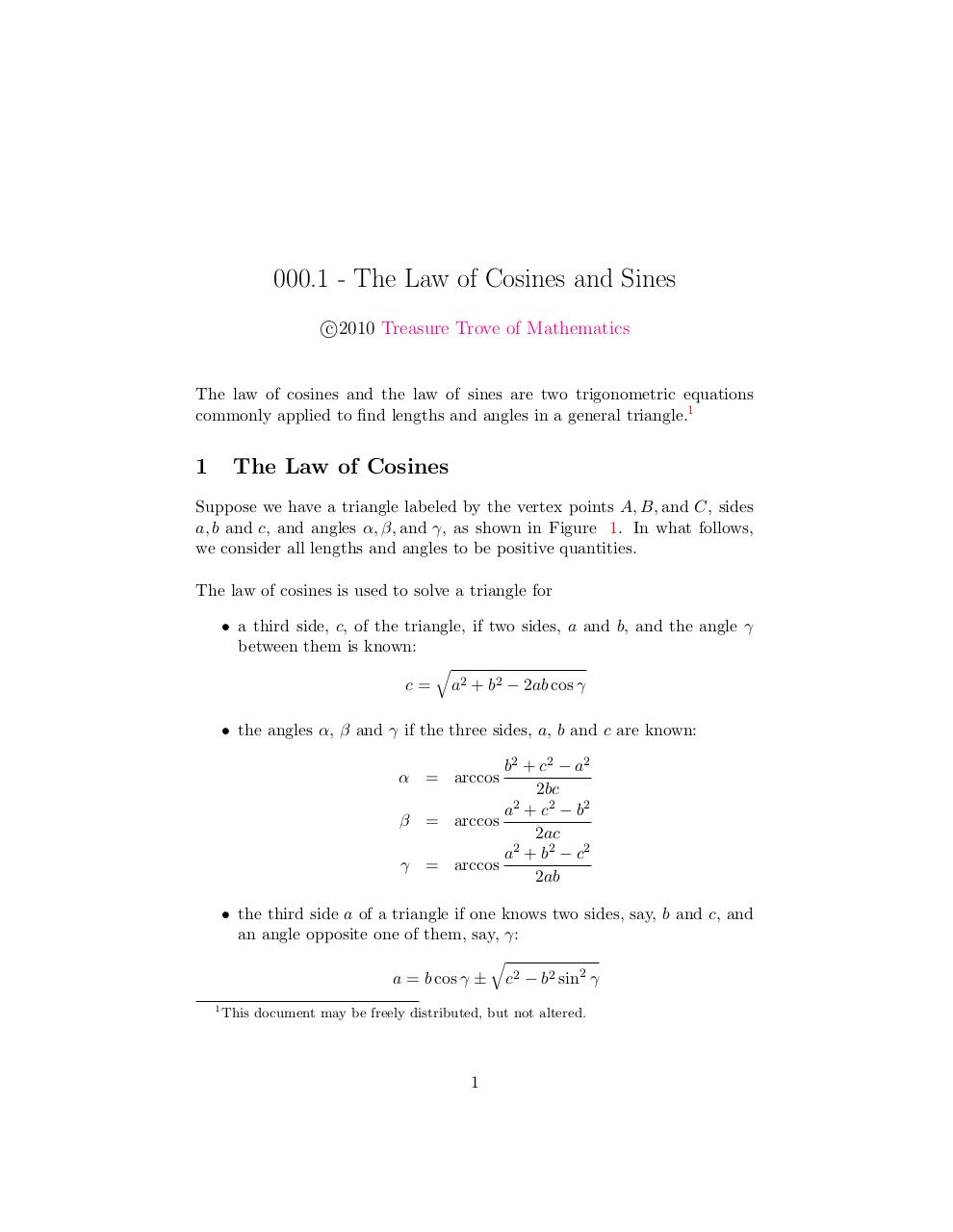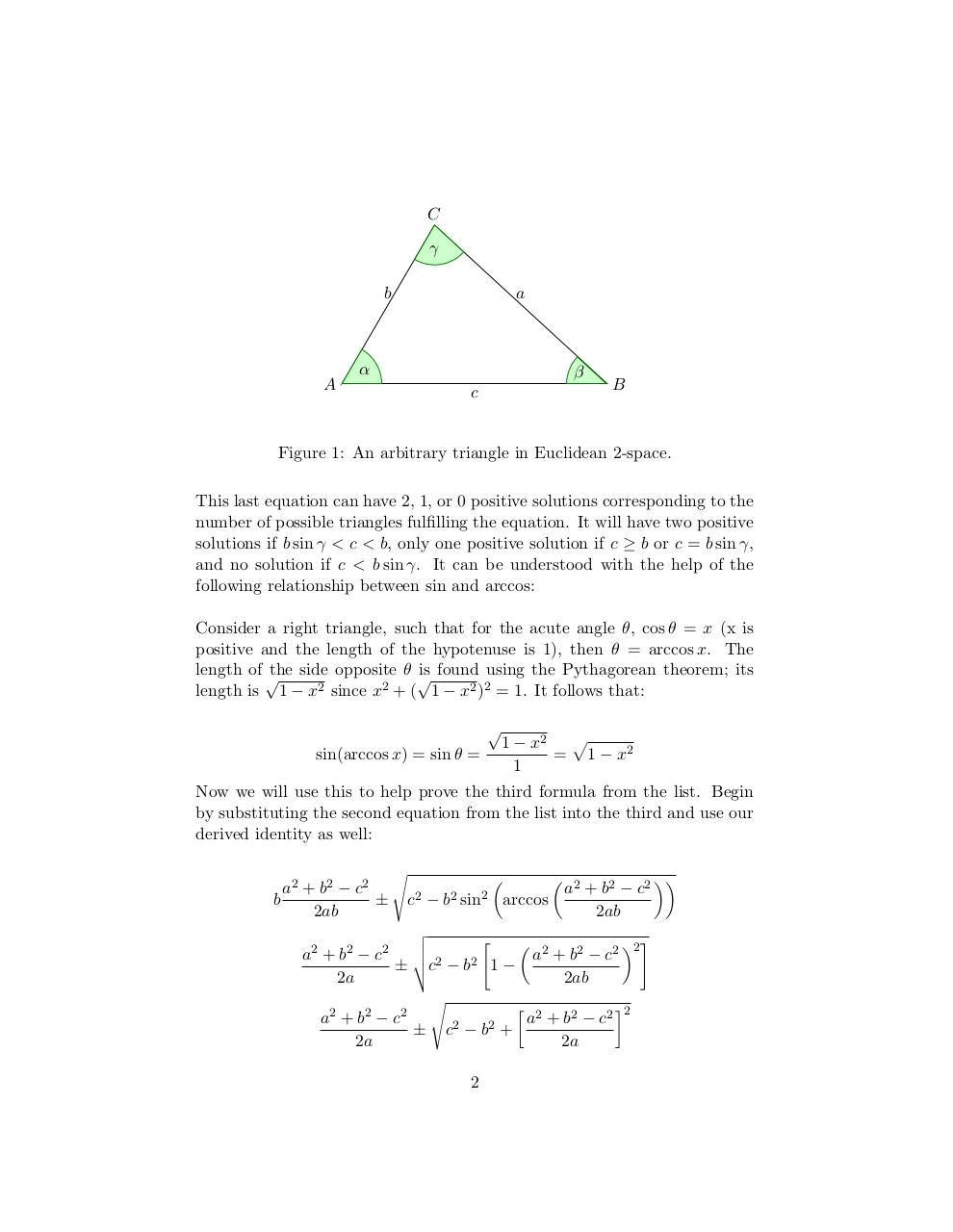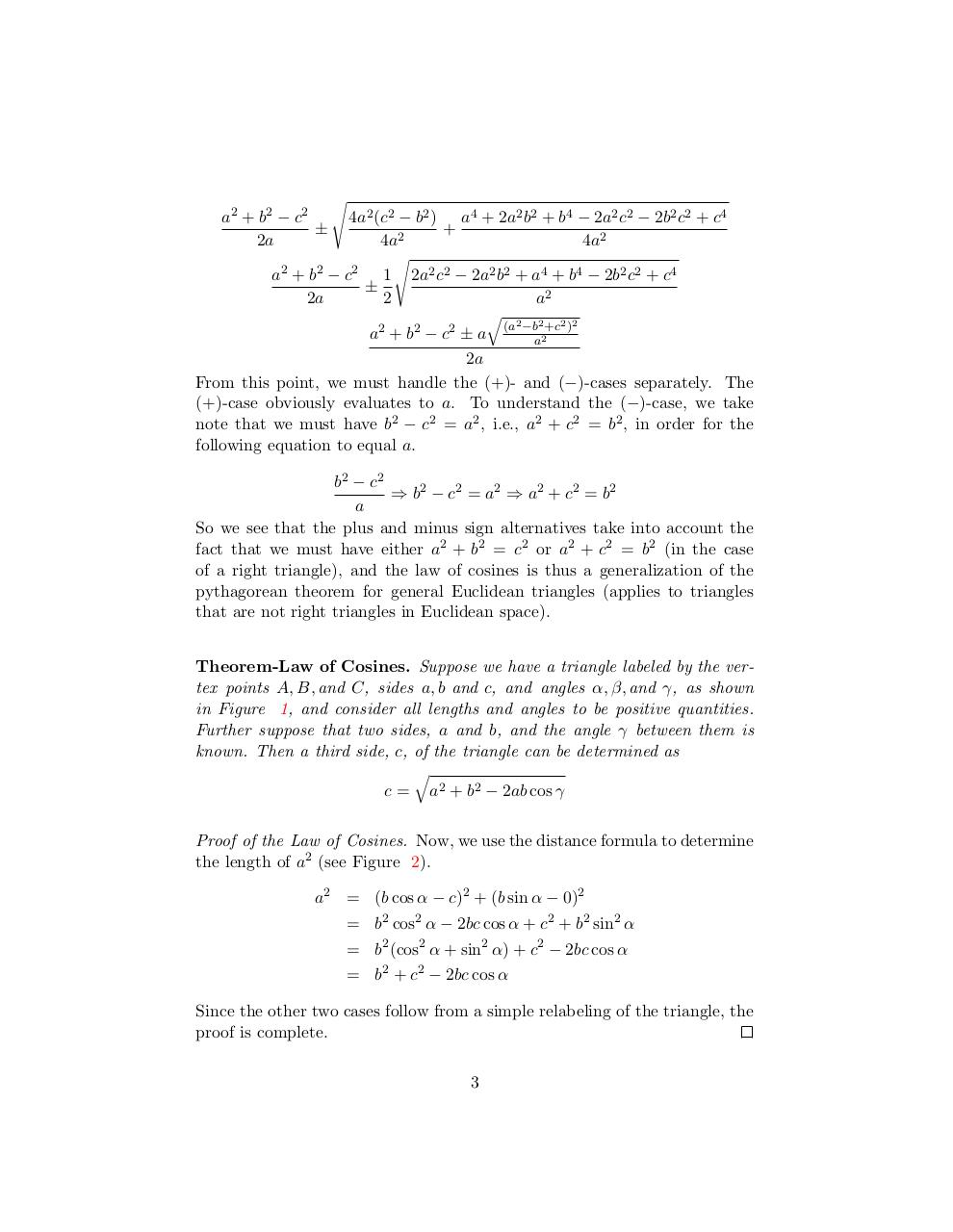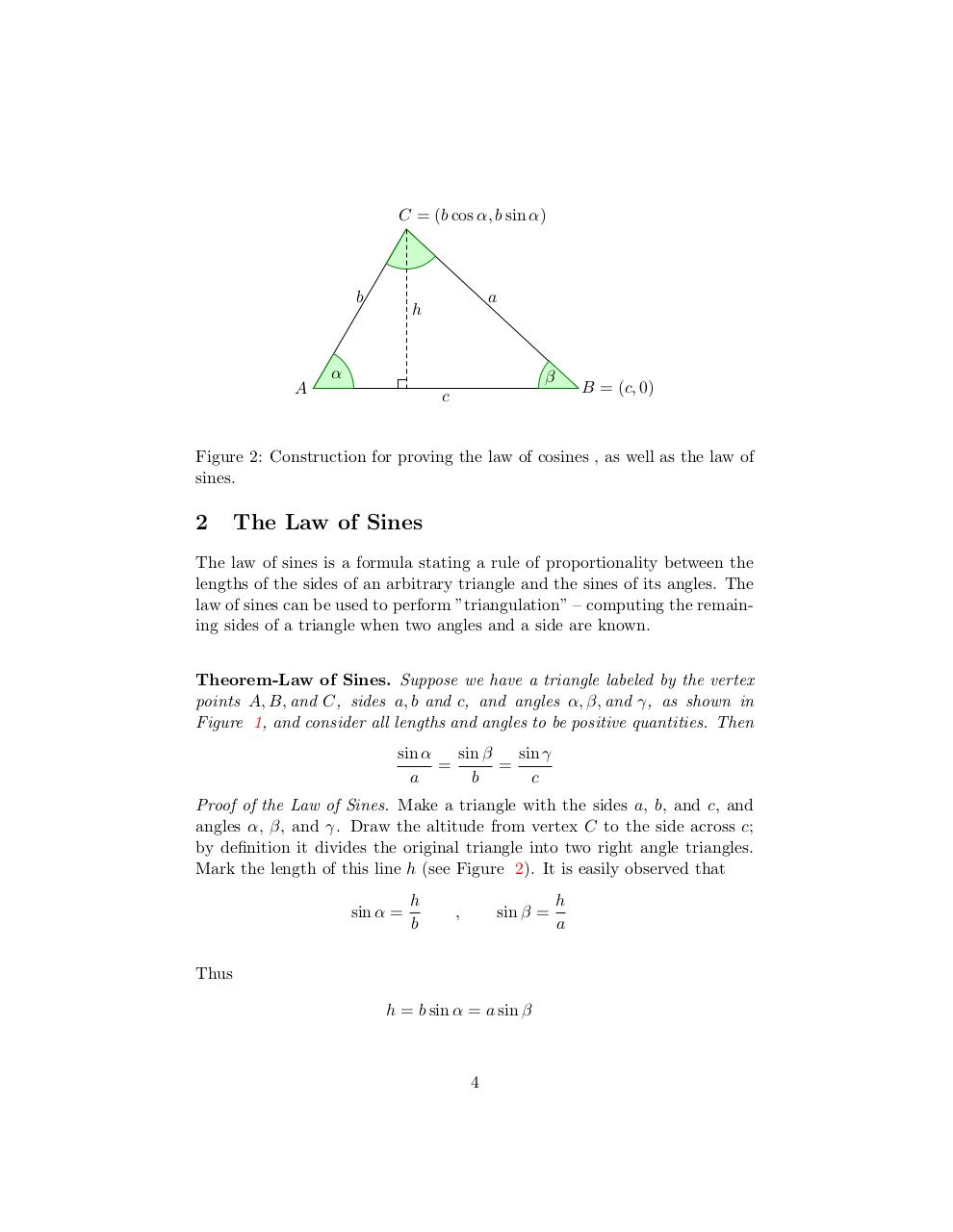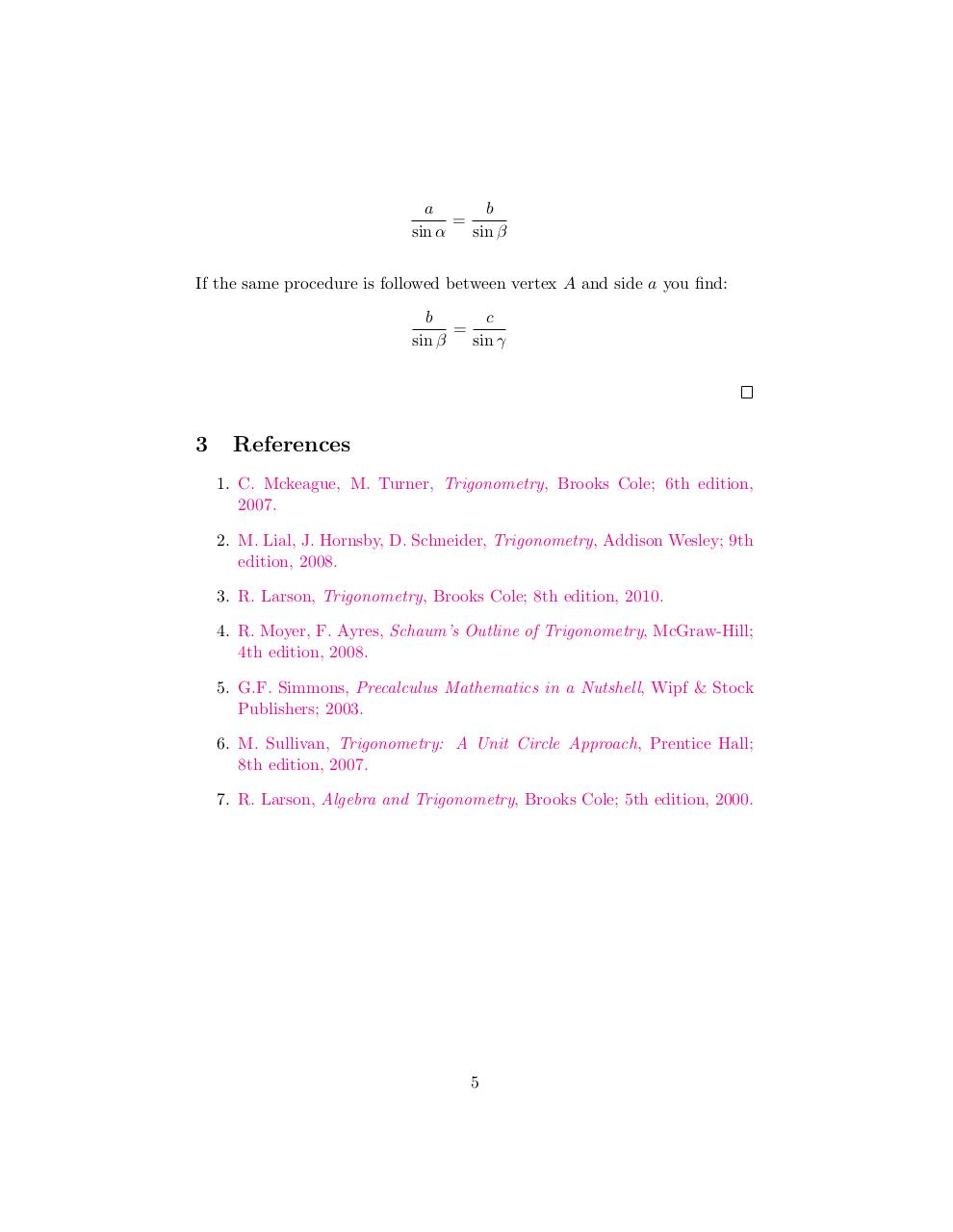#### HTML Code

Copy the following HTML code to share your document on a Website or Blog

#### QR Code### Related keywords